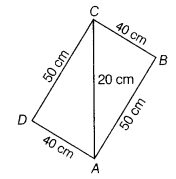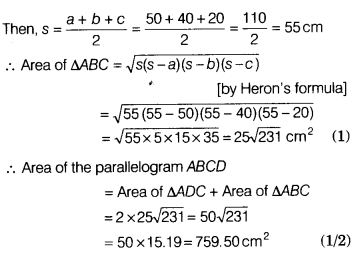# The lengths of two adjacent sides of a parallelogram are respectively 40 cm and 50 The lengths of two adjacent sides of a parallelogram are respectively 40 cm and 50 cm

The lengths of two adjacent sides of a parallelogram are respectively 40 cm and 50 cm. One of its diagonals is 20 cm. Find the area of the parallelogram.

Let ABCD be the given parallelogram with AB=50 cm, BC = 40 cm and diagonal AC = 20 cm.
Now, in ∆ABC,
a = 40 cm, b = 20 cm and c = 50 cm